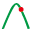#### File Exchange > Data Analysis >    Power and Sample Size for Equivalence TestsAuthor:
OriginLab Technical Support
12/15/2022
Last Update:
4/27/2023
61
Total Ratings:
0
File Size:
200 KB
Average Rating:File Name:
Power_and_...ts.opx
File Version:
1.00
Minimum Versions:
Summary:

Perform power and sample size analysis for equivalence tests including one-sample, two-sample and paired-sample.

Description:

PURPOSE
This app can be used to calculate power and sample size for equivalence tests, including one-sample, two-sample and paired.

INSTALLATION
Download the file Power_and_Sample_Size_for_Equivalence_Tests.opx, and then drag-and-drop onto the Origin workspace. An icon will appear in the Apps Gallery window.
NOTE: This tool requires OriginPro.

OPERATION

1. Make a worksheet active, Click Power and Sample Size for Equivalence Tests icon in the Apps Gallery window. A toolbar will appear with three buttons: Power and Sample Size for One-Sample Equivalence TestPower and Sample Size for Two-Sample Equivalence Test, and Power and Sample Size for Paired Equivalence Test.
2. Click Power and Sample Size for One-Sample Equivalence Test button, a dialog will open.
1. Calculate drop-down list has two options: Power and Sample Size, and it determines which quantity to calculate.
2. In Hypothesis group, Alternative Hypothesis includes three options: Mean - Target > Lower LimitMean - Target > Upper Limit, and Lower Limit < Mean - Target < Upper Limit. Values for Lower Limit and Upper Limit are specified in the edit boxes.
3. In Test Specification branch, settings include DifferenceStandard Deviation and Alpha. If Power is chosen, Hypothetical Sample Size(s) edit box supports multiple sample sizes separated with space. Otherwise, Hypothetical Power(s) edit box is shown.
4. In Options branch, Check More Hypothetical Sample Size(s) (Calculate = Power) check box, Hypothetical Sample Size(s) Column will be enabled. It allows you to specify sample size(s) in a column. Check Show Power Curves, it can create power vs difference curves for different sizes. If it fails to find the sample size, check Search One by One (Calculate = Sample Size) option, and this method can find the target, but it is very slow. Maximum Iterations can be used to control iteration of cumulative distribution function for non-central t distribution.
3. Click Power and Sample Size for Two-Sample Equivalence Test button, the dialog is similar to Power and Sample Size for One-Sample Equivalence Test dialog.
1. In Hypothesis group, it includes a Type drop-down list: Difference = Mean - Target and Ratio = Mean / Target.
2. In Test Specification branch, when Ratio is chosen in Type drop-down list, Difference and Standard Deviation are replaced with Ratio and Coefficient of Variation (CV) respectively.
4. Click Power and Sample Size for Paired Equivalence Test button, the dialog is similar to Power and Sample Size for Two-Sample Equivalence Test dialog. The only difference is that in Test Specification branch, when Ratio is chosen in Type drop-down list, Coefficient of Variation (CV) edit box is replaced with Standard Deviation of Paired Differences (Log Transformed).

SAMPLE OPJU FILE
This app provides a sample OPJU file. Right click on the Power and Sample Size for Equivalence Tests icon in the Apps Gallery window, and choose Show Samples Folder from the short-cut menu. A folder will open. Drag-and-drop the project file PSSEqTestSample.opju from the folder onto Origin. The Notes window in the project shows detailed steps. And results are shown in Book1.
Note: If you wish to save the OPJU after changing, it is recommended that you save to a different folder location (e.g. User Files Folder).

ALGORITHM

• Power and Sample Size for One-Sample Equivalence Test

For sample size of n, degrees of freedom $$\nu = n - 1$$, the upper $$\alpha$$ critical value for t-distribution is denoted by t. The power can be calculated by the noncentral Student's t-distribution.

1. H1: Mean - Target > Lower Limit

Noncentrality parameter is:

$$\delta_1 = \frac{ D - l }{ \sigma/ \sqrt{n}}$$, where D is the difference, l is the lower limit, and $$\sigma$$is the standard deviation.

The power is: $$P = 1 - CDF( t: \nu, \delta_1 )$$, where CDF is the cumulative function for the noncentral Student's t-distribution.

2. H1: Mean - Target < Upper Limit

$$\delta_2 = \frac{ D - u }{ \sigma / \sqrt{n} }$$, where u is the upper limit.

The power is: $$P = CDF( -t: \nu, \delta_2 )$$

3. H1: Lower Limit < Mean - Target < Upper Limit

The power is: $$P = CDF( -t: \nu, \delta_2 ) - CDF( t: \nu, \delta_1 )$$

• Power and Sample Size for Two-Sample Equivalence Test

It is similar to one sample test except that $$\nu = 2n-2$$, and $$\delta_1 = \frac{ D - l }{ \sigma \sqrt{2/n}}$$$$\delta_2 = \frac{ D - u }{ \sigma \sqrt{2/n}}$$, where n is sample size of two samples.

If power is calculated for ratio, perform log transformation first for lower/upper limit and ratio. The standard deviation is: $$\sigma = \sqrt{ \ln( 1+CV^2 ) }$$, and CV is the coefficient of variation.

• Power and Sample Size for Paired Equivalence Test

It is similar to one sample test except that n is the sample size of paired samples, and $$\sigma$$ is the standard deviation of the difference between paired samples.

If power is calculated for ratio, perform log transformation first for lower/upper limit and ratio, and $$\sigma$$ is the standard deviation of the difference between log transformed paired samples.

Related Apps